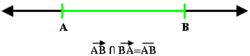# Line segment

Line segmentThe geometric definition of a line segment

In geometry, a line segment is a part of a line that is bounded by two end points, and contains every point on the line between its end points. Examples of line segments include the sides of a triangle or square. More generally, when the end points are both vertices of a polygon, the line segment is either an edge (of that polygon) if they are adjacent vertices, or otherwise a diagonal. When the end points both lie on a curve such as a circle, a line segment is called a chord (of that curve).

## Definition

If$V\,\!$ is a vector space over$\mathbb{R}$ or$\mathbb{C}$, and$L\,\!$ is a subset of$V,\,\!$ then$L\,\!$ is a line segment if$L\,\!$ can be parameterized as$L = \{ \mathbf{u}+t\mathbf{v} \mid t\in[0,1]\}$

for some vectors$\mathbf{u}, \mathbf{v} \in V\,\!$, in which case the vectors$\mathbf{u}$ and$\mathbf{u+v}$ are called the end points of$L.\,\!$

Sometimes one needs to distinguish between "open" and "closed" line segments. Then one defines a closed line segment as above, and an open line segment as a subset$L\,\!$ that can be parametrized as$L = \{ \mathbf{u}+t\mathbf{v} \mid t\in(0,1)\}$

for some vectors$\mathbf{u}, \mathbf{v} \in V\,\!$.

Equivalently, a line segment is the convex hull of two points. Thus, the line segment can be expressed as a convex combination of the segment's two end points.

In geometry, it is sometimes defined that a point B is between two other points A and C, if the distance AB added to the distance BC is equal to the distance AC. Thus the equation of a line segment with endpoints A =(ax,ay) and C =(cx,cy) is$\sqrt{(x-c_x)^2 + (y-c_y)^2} + \sqrt{(x-a_x)^2 + (y-a_y)^2} = \sqrt{(c_x-a_x)^2 + (c_y-a_y)^2}.$

## In proofs

In an axiomatic treatment of Geometry, the notion of betweenness is either assumed to satisfy a certain number of axioms, or else defined in terms of an isometry of a line (used as a coordinate system).

Segments play an important role in other theories. For example, a set is convex if the segment that joins any two points of the set is contained in the set. This is important because it transforms some of the analysis of convex sets to the analysis of a line segment.

## As a degenerate ellipse

A line segment can be viewed as a degenerate case of an ellipse in which the semiminor axis goes to zero, the foci go to the end points, and the eccentricity goes to one. As a degenerate orbit this is a radial elliptic trajectory.

Wikimedia Foundation. 2010.

### Look at other dictionaries:

• line segment — Geom. segment (def. 2b). * * * line segment, any finite section between two points on a line; segment …   Useful english dictionary

• Line segment intersection — In computational geometry, the line segment intersection problem supplies a list of line segments in the plane and asks us to determine whether any two of them intersect, or cross.Naive algorithms examine each pair of segments, but this is highly …   Wikipedia

• line segment — noun A part of a straight line bounded by two <!redundant: end points.<!redundant; this is implicit in the fact that this is a line: and contains every point on the line between its end points …   Wiktionary

• line segment — Geom. segment (def. 2b). * * * …   Universalium

• line segment —    In vector graphics, same as vector …   IT glossary of terms, acronyms and abbreviations

• Segment — may mean: *an internal section of a citrus fruit *Market segment, the smaller subgroups comprising a marketComputing *Memory segment, the portion of computer memory which may be addressed by a single index register *Image segment created in… …   Wikipedia

• Line-intercept sampling — (LIS) is a method of sampling elements in a region whereby an element is sampled if a chosen line segment, called a “transect”, intersects the element Kaiser, L, 1983. Unbiased Estimation in Line Intercept Sampling, Biometrics 39. pp 965 976. ] …   Wikipedia

• Line (geometry) — Three lines the red and blue lines have the same slope, while the red and green ones have same y intercept …   Wikipedia

• line — 1. noun /laɪn/ a) A path through two or more points (compare ‘segment’); a continuous mark, including as made by a pen; any path, curved or straight. <! should this be split into two senses: path and mark ? The atmosphere in flaming sparkles… …   Wiktionary

• segment — segmentary /seg meuhn ter ee/, adj. segmentate, adj. n. /seg meuhnt/; v. /seg ment, seg ment /, n. 1. one of the parts into which something naturally separates or is divided; a division, portion, or section: a segment of an orange. 2. Geom. a. a… …   Universalium